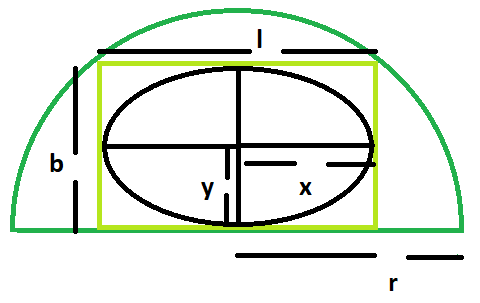# Largest ellipse that can be inscribed within a rectangle which in turn is inscribed within a semicircle

Given here is a semicircle of radius r, which inscribes a rectangle which in turn inscribes an ellipse. The task is to find the area of this largest ellipse.

Examples:

```Input: r = 5
Output: 19.625

Input: r = 11
Output: 94.985
```Approach:

1. Let the, length of the rectangle = l and breadth of the rectangle = b
2. Let, the length of the major axis of the ellipse = 2x and, the length of the minor axis of the ellipse = 2y
3. As we know, length and breadth of the largest rectangle inside a semicircle are r/√2 and √2r(Please refer here)
4. Also, Area of the ellipse within the rectangle = (π*l*b)/4 = (πr^2/4)

Below is the implementation of above approach:

## C++

 `// C++ Program to find the biggest ellipse ` `// which can be inscribed within a rectangle ` `// which in turn is inscribed within a semicircle ` ` `  `#include ` `using` `namespace` `std; ` ` `  `// Function to find the area ` `// of the biggest ellipse ` `float` `ellipsearea(``float` `r) ` `{ ` ` `  `    ``// the radius cannot be negative ` `    ``if` `(r < 0) ` `        ``return` `-1; ` ` `  `    ``// area of the ellipse ` `    ``float` `a = (3.14 * r * r) / 4; ` ` `  `    ``return` `a; ` `} ` ` `  `// Driver code ` `int` `main() ` `{ ` `    ``float` `r = 5; ` `    ``cout << ellipsearea(r) << endl; ` `    ``return` `0; ` `} `

## Java

 `// Java Program to find the biggest ellipse ` `// which can be inscribed within a rectangle ` `// which in turn is inscribed within a semicircle ` `class` `GFG ` `{ ` ` `  `// Function to find the area ` `// of the biggest ellipse ` `static` `float` `ellipsearea(``float` `r) ` `{ ` ` `  `    ``// the radius cannot be negative ` `    ``if` `(r < ``0``) ` `        ``return` `-``1``; ` ` `  `    ``// area of the ellipse ` `    ``float` `a = (``float``)((``3``.14f * r * r) / ``4``); ` ` `  `    ``return` `a; ` `} ` ` `  `// Driver code ` `public` `static` `void` `main(String[] args) ` `{ ` `    ``float` `r = ``5``; ` `    ``System.out.println(ellipsearea(r)); ` `} ` `} ` ` `  `// This code is contributed by Code_Mech. `

## Python3

 `# Python3 Program to find the biggest ellipse  ` `# which can be inscribed within a rectangle  ` `# which in turn is inscribed within a semicircle  ` ` `  `# Function to find the area of  ` `# the biggest ellipse  ` `def` `ellipsearea(r) :  ` ` `  `    ``# the radius cannot be negative  ` `    ``if` `(r < ``0``) : ` `        ``return` `-``1``;  ` ` `  `    ``# area of the ellipse  ` `    ``a ``=` `(``3.14` `*` `r ``*` `r) ``/` `4``;  ` ` `  `    ``return` `a;  ` ` `  `# Driver code  ` `if` `__name__ ``=``=` `"__main__"` `: ` ` `  `    ``r ``=` `5``;  ` `    ``print``(ellipsearea(r));  ` ` `  `# This code is contributed by Ryuga `

## C#

 `// C# Program to find the biggest ellipse ` `// which can be inscribed within a rectangle ` `// which in turn is inscribed within a semicircle ` `using` `System; ` `class` `GFG ` `{ ` ` `  `// Function to find the area ` `// of the biggest ellipse ` `static` `float` `ellipsearea(``float` `r) ` `{ ` ` `  `    ``// the radius cannot be negative ` `    ``if` `(r < 0) ` `        ``return` `-1; ` ` `  `    ``// area of the ellipse ` `    ``float` `a = (``float``)((3.14 * r * r) / 4); ` ` `  `    ``return` `a; ` `} ` ` `  `// Driver code ` `public` `static` `void` `Main() ` `{ ` `    ``float` `r = 5; ` `    ``Console.WriteLine(ellipsearea(r)); ` `} ` `} ` ` `  `// This code is contributed by Akanksha Rai `

## PHP

 ` `

Output:

```19.625
```

Attention reader! Don’t stop learning now. Get hold of all the important DSA concepts with the DSA Self Paced Course at a student-friendly price and become industry ready.

My Personal Notes arrow_drop_upCheck out this Author's contributed articles.

If you like GeeksforGeeks and would like to contribute, you can also write an article using contribute.geeksforgeeks.org or mail your article to contribute@geeksforgeeks.org. See your article appearing on the GeeksforGeeks main page and help other Geeks.

Please Improve this article if you find anything incorrect by clicking on the "Improve Article" button below.

Article Tags :
Practice Tags :

Be the First to upvote.

Please write to us at contribute@geeksforgeeks.org to report any issue with the above content.